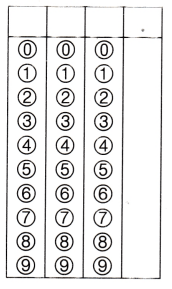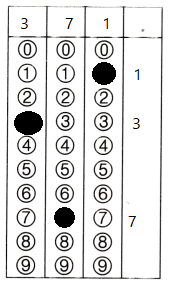Refer to our Texas Go Math Grade 3 Answer Key Pdf to score good marks in the exams. Test yourself by practicing the problems from Texas Go Math Grade 3 Module 10 Assessment Answer Key.

Vocabulary

Choose the best term from the box to complete the sentence.

Question 1.
The ___ is the number that is to be divided in a division problem. (p. 320)
Explanation:
A number that divides another number with or without leaving a remainder is called a divisor.

You ___ when you separate into equal groups.
Explanation:
To separate into two or more parts or pieces She divided the pie into eight pieces.

Concepts and Skills

Use counters or draw a quick picture on your MathBoard. Make or circle equal groups. Complete the table. TEKS 3.4.H, 3.4.K.Explanation:
Completed the table.
By filling the table with the counters.

Make an array. Then write a division equation. TEKS 3.4.H, 3.4.K,

Question 6.
32 tiles in 4 rows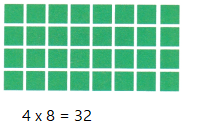Explanation:32 ÷  4 = 8
An array is shown to solve the problem

28 tiles in 7 rows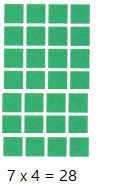Explanation:
28 ÷ 7 = 4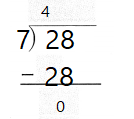An array is shown to solve the problem

Write a division equation. TEKS 3.4.H, 3.4.K

Question 8.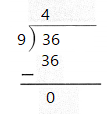Explanation:
The division equation is written for 36 divided by 9

Question 9.21 ÷  3 = 7
Explanation: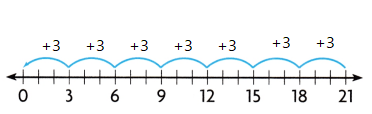Texas Test prep

Fill in the bubble for the correct answer choice.
You may use objects or models to solve.

Question 10.
Desiree has 20 stickers. She gives the same number of stickers to each of 3 friends and 2 of her sisters. Which equation can be used to find the number of stickers each person receives? TEKS 3.4.H, 3.4.K, 3.5.B
(A) 20 ÷ 2 = ___
(B) 20 × 5 = ___
(C) 20 – 5 = ___
(D) 20 ÷ 5 = ___
Explanation:
20 ÷ 5 = 4
she gave 4 stickers to each person.

Texas Go Math Grade 3 Answer Key Pdf Module 10 Question 11.
Which division equation does the array show? TEKS 3.4.H, 3.4.K, 3.5.B
(A) 40 ÷ 5 = 8
(B) 8 ÷ 40 = 5
(C) 32 ÷ 4 = 8
(D) 40 ÷ 4 = 10Explanation:
40 ÷ 5 = 8 the array shows

Question 12.
Lillian bought 24 cans of cat food. There were 4 cans in each pack. How many packs of cat food did Lillian buy? TEKS 3.4.H, 3.4.K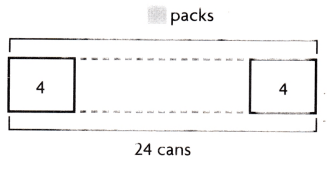(A) 7
(B) 6
(C) 5
(D) 8
6 x 4 = 24
Lillian buy 6 packs of cat food